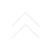## Elastic moduli measurement system

### Measurement of porous material elastic moduli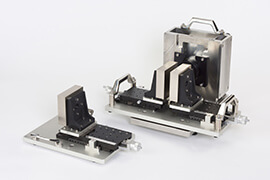Nihon Onkyo Engineering provides a system that measures elastic moduli, Young's modulus and shear modulus that characterize the acoustic property of poro-elastic material. These parameters can be used in STRATI-ARTZ acoustic material modeling software and in various simulation software using SEA or FEM calculations.

### What is porous material elasticity?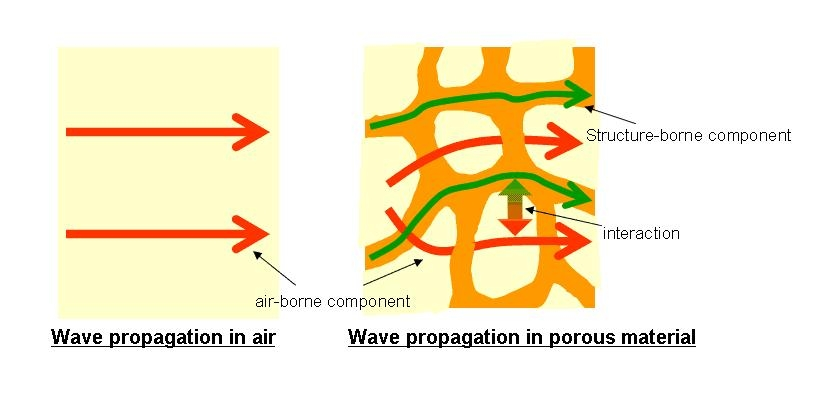Although it may be difficult to imagine compared with solid elastic material like rubber, the Biot model takes into consideration both the air-borne sound in the porous material and the structure-borne sound propagating through the frame of the material. The elastic moduli are related to the structure-borne component. This component can be ignored for single-layered fibrous material but cannot be ignored for the material like urethane foam. Moreover, when using STRATI-ARTZ software to create an acoustic model for predicting the characteristics of a multi-layered structure, these parameters should be indispensable even if the material is fibrous.
There are two kinds, Young's modulus and the shear modulus. From the measured these two parameters, Poisson's ratio can be determined.

### Measurement of Young's modulus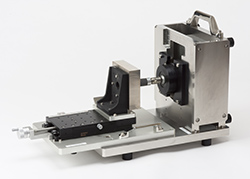When measuring Young's modulus, the measurement sample is set on a shaker, and then a weight is placed on top. Random vertical vibration is applied to measure the vibration transmission characteristics. The frequency characteristics of this vibration transfer are used to determine the Young's modulus and the attenuation coefficient. The attenuation coefficient sometimes called as loss factor.

### Measurement of shear modulus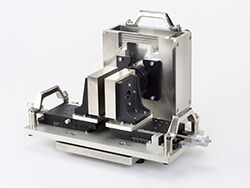Shear modulus measurement is similar to measurement of Young's modulus except with shear force (displacement force) applied to the sample. Nihon Onkyo Engineering's system provides a shear force by sandwiching the vibrating plate between two sample sheets. As with Young's modulus measurement, the modulus and attenuation coefficient are calculated from the vibration transfer frequency response.